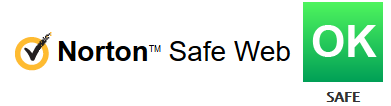# Multiplication Table Printable

## Enregistrer, Remplir les champs vides, Imprimer, Terminer!How to memorize multiplication tables? How do you make an multiplication table? Download this multiplication table template that will perfectly suit your needs!

Formats de fichiers gratuits disponibles:

.xlsx

• Ce document a été certifié par un professionnel
• 100% personnalisable

Évaluation du modèle: 8

Aucun Malware/Virus trouvé, scanné par:Education Éducation math mathematics mathématiques multiply multiplier multiplication table table de multiplication math multiply multiplier les maths math conversion conversion mathématique multiplication tables tables de multiplication printable multiplication table animated math cool math elementary math powerpoint templates eureka math templates everyday math templates free jamboard math templates free mathematics powerpoint templates free ppt templates math theme free website templates for mathematics goodnotes math templates google docs math templates jamboard math templates latex math templates math binder cover templates math graphic organizer templates math hyperdoc templates math interactive notebook templates math jamboard templates math powerpoint templates math progress monitoring templates math templates excel math templates for grade math templates for kindergarten math templates for teachers math templates free download math themed template math worksheet mathematics certificates mathematics formulas math templates maths templates free microsoft powerpoint templates for mathematics microsoft word math templates powerpoint math templates multiplication table printable multiplication table chart 12 multiplication table multiplication table up to 100 multiplication table up to printable multiplication chart memorize multiplication tables multiplication table 12 multiplication tables to 20

How do you make an multiplication table? Download this multiplication table template that will perfectly suit your needs!

Mathematicians regularly need spreadsheets, worksheets, or forms, and often more than any other industry. Therefore, we support you by providing this multiplication table pdf template, which will save your time and effort and help you to reach the next level of success in your studies, work, or business!

How to memorize multiplication tables?

In math, the multiplication table is a a common mathematical table that is useful to memorize the tables, often from 1 to 12 for children. It defines the multiplication operation for an algebraic system. Often math templates are used to make calculations, such as conversions (Temperature Conversion, Weight Conversion, and so on). By using a clean and modern-looking math template from AllBusinessTemplates, you know you are already halfway on the road to success. Although there have been many different measurements and the definitions of the units have been revised, the official system of measurements of most countries is the modern form of the metric system which is known as the "International System of Units".

This multiplication table pdf is intuitive, ready-to-use, and structured in a smart way. Try it now and let this mathematics template inspire you. We certainly encourage you to use this multiplication table pdf for your own good and are confident it will fit your needs. Our site is often updated with new multiplication and educational templates. Use modern and high-quality professional math templates to solve issues or create amazingly clear presentations, when you work in education, Physics, Chemistry, etc.

AVERTISSEMENT
Rien sur ce site ne doit être considéré comme un avis juridique et aucune relation avocat-client n'est établie.

Si vous avez des questions ou des commentaires, n'hésitez pas à les poster ci-dessous.Voir plus